Open in App
Not now

# Relative and Absolute Cell References in MS Excel

• Difficulty Level : Basic
• Last Updated : 06 Jun, 2021

Cell reference is the address or name of a cell or a range of cells. It is the combination of column name and row number. It helps the software to identify the cell from where the data/value is to be used in the formula. We can reference the cell of other worksheets and also of other programs.

• Referencing the cell of other worksheets is known as External referencing.
• Referencing the cell of other programs is known as Remote referencing.

There are two types of cell references in Excel:

• Relative reference
• Absolute reference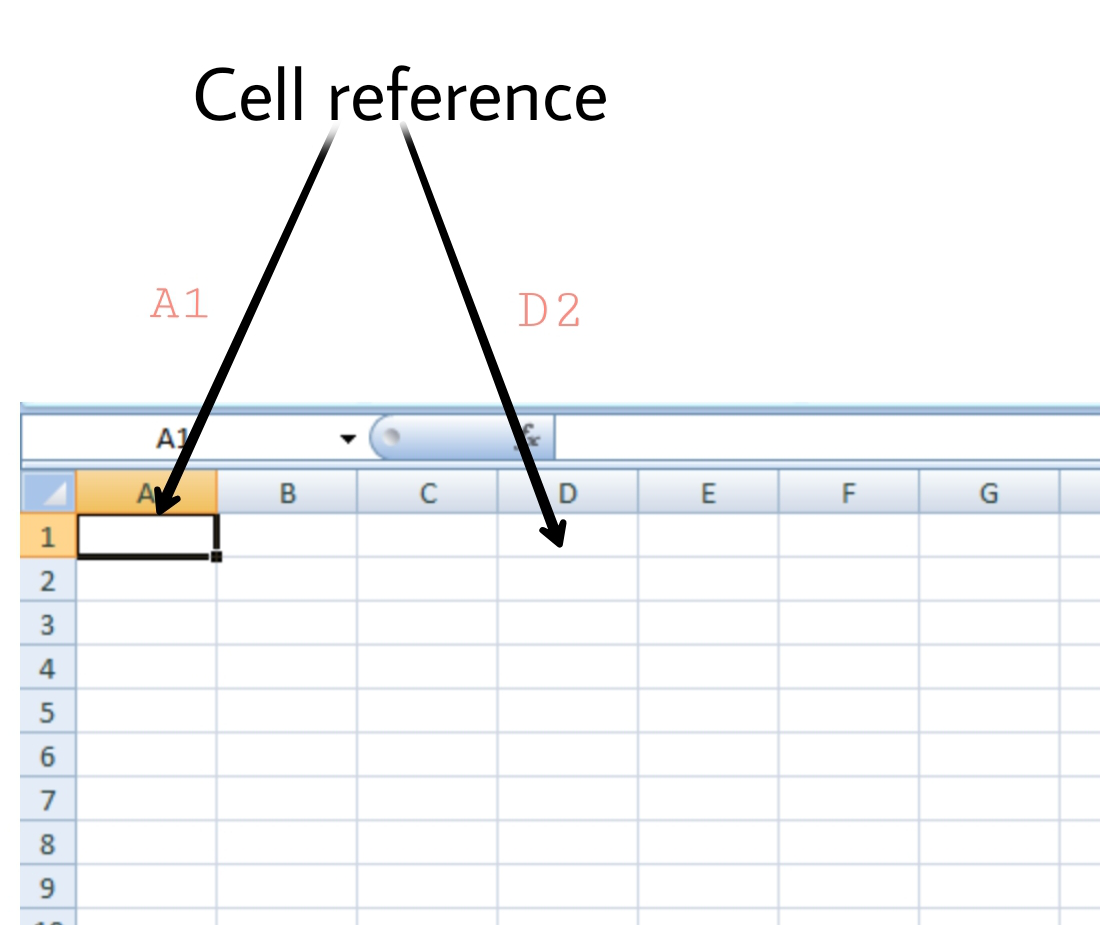### Relative Reference

Relative reference is the default cell reference in Excel. It is simply the combination of column name and row number without any dollar (\$) sign. When you copy the formula from one cell to another the relative cell address changes depending on the relative position of column and row. C1, D2, E4, etc are examples of relative cell references. Relative references are used when we want to perform a similar operation on multiple cells and the formula must change according to the relative address of column and row.

For example, We want to add the marks of two subjects entered in column A and column B and display the result in column C. Here, we will use relative reference so that the same rows of column’s A and B are added.

Steps to Use Relative Reference:

Step 1: We write the formula in any cell and press enter so that it is calculated. In this example, we write the formula(= B2 + A2) in cell C2 and press enter to calculate the formula.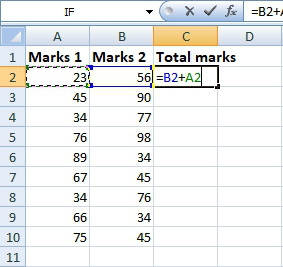Step 2: Now click on the Fill handle at the corner of cell which contains the formula(C2).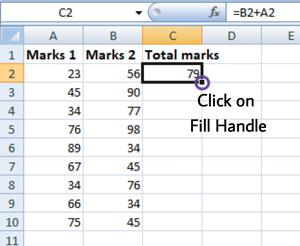Step 3: Drag the Fill handle up to the cells you want to fill. In our example, we will drag it till cell C10.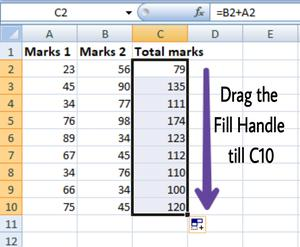Step 4: Now we can see that the addition operation is performed between the cell A2 and B2, A3 and B3 and so on.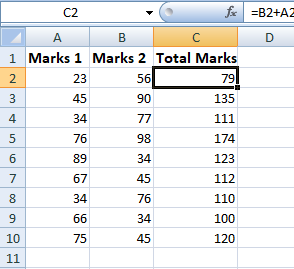Step 5: You can double-click on any cell to check that the operation is performed in between which cells.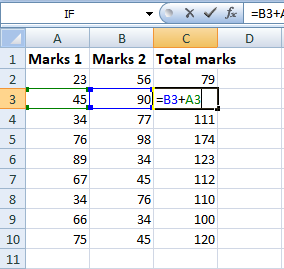Thus, in the above example, we see that the relative address of cell A2 changes to A3, A4, and so on, similarly the relative address changes for column B, depending on the relative position of the row.

### Absolute Reference

Absolute reference is the cell reference in which the row and column are made constant by adding the dollar (\$) sign before the column name and row number. The absolute reference does not change as you copy the formula from one cell to other. If either the row or the column is made constant then it is known as a mixed reference. You can also press the F4 key to make any cell reference constant. \$A\$1, \$B\$3 are examples of absolute cell reference.

For example, We want to multiply the sum of marks of two subjects, entered in column A and column B, with the percentage entered in cell C2 and display the result in column D. Here, we will use absolute reference so that the address of cell C2 remains constant and does not change with the relative position of column and rows.

Steps to Use Absolute Reference:

Step 1: We write the formula in any cell and press enter so that it is calculated. In this example, we write the formula(=(A2+B2)*\$C\$2) in cell D2 and press enter to calculate the formula.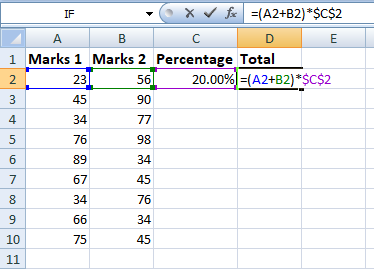Step 2: Now click on the Fill handle at the corner of cell which contains the formula(D2).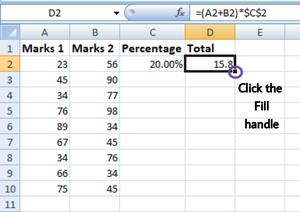Step 3: Drag the Fill handle up to the cells you want to fill. In our example, we will drag it till cell D10.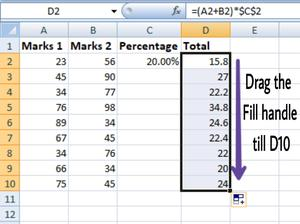Step 4: Now we can see that the percentage is calculated in column D.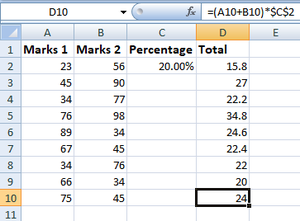Step 5: You can double-click on any cell to check that the operation is performed in between which cells, and we see that the address of cell C2 does not change.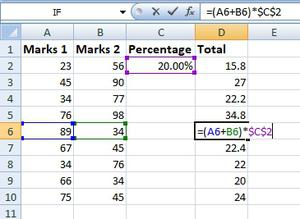Thus, in the above example we see that the address of cell C2 is not changed whereas the address of column A and B changes with the relative position of the row and column, this happened because we used the absolute address of the cell C2.

My Personal Notes arrow_drop_up